多元函数

向量值函数（Vector-valued Functions or Vector Functions）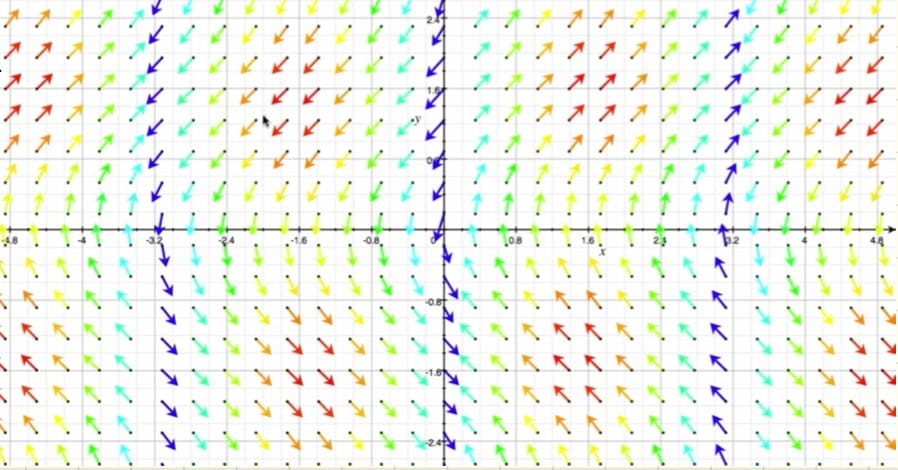可视化（Visualization）

1. 图形：通常只适用于二对一的函数，如 $f(x,y)=e^{-(x^2+y^2)}$、$f(x,y)=\cos(x)\sin(y)$。

在实际中，对于维度较高的函数如 $f(x,y,z)=x^2+y^2+z^2$，人们通常先研究 $f(x,y)=x^2+y^2$ 的性质（概念原型），并将发现的一些性质推广到原始的高维函数中。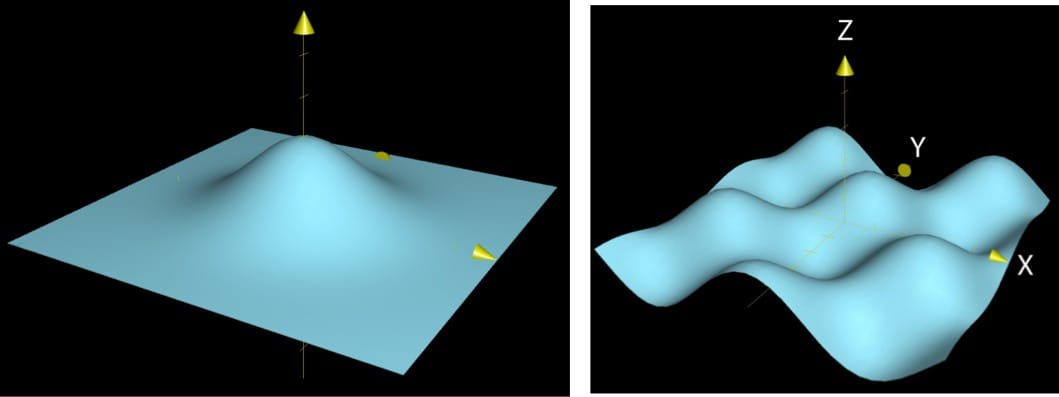2. 等高线图：该图只显示输入空间，适用于二对一的函数。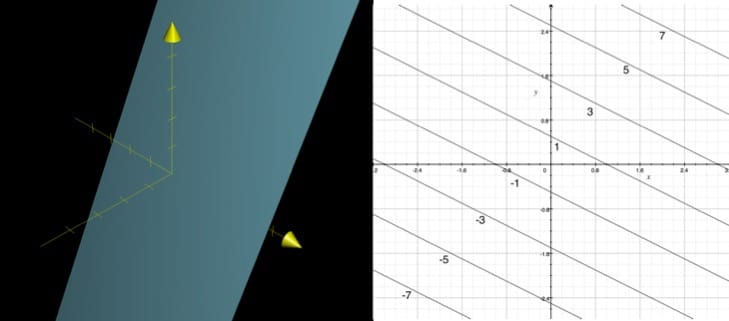3. 参数曲线/平面：适用于输出空间维度大于输入空间的情况，如 f(t)=\left[\begin{aligned}2t\\3t \end{aligned}\right]、f(t)=\left[\begin{aligned}\sin&(t)\\\cos&(3t)\\3&t \end{aligned}\right]。

• 一个输入：f(t)=\left[\begin{aligned}t\cos(2\pi t)\\t\sin(2\pi t)\end{aligned}\right]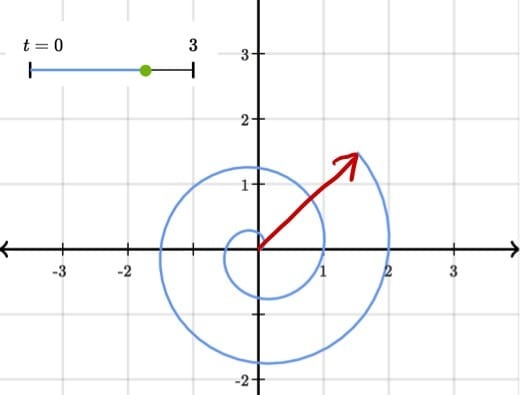• 两个输入：f(s,t)=\left[\begin{aligned}t^3&-st\\s&-t\\s&+t\end{aligned}\right]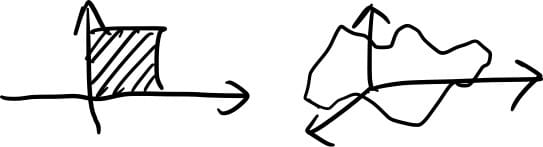4. 向量场：适用于输入空间维度与输出空间维度相等的函数，如 f(x,y)=\left[\begin{aligned}&1\\y^2&-y\end{aligned}\right]。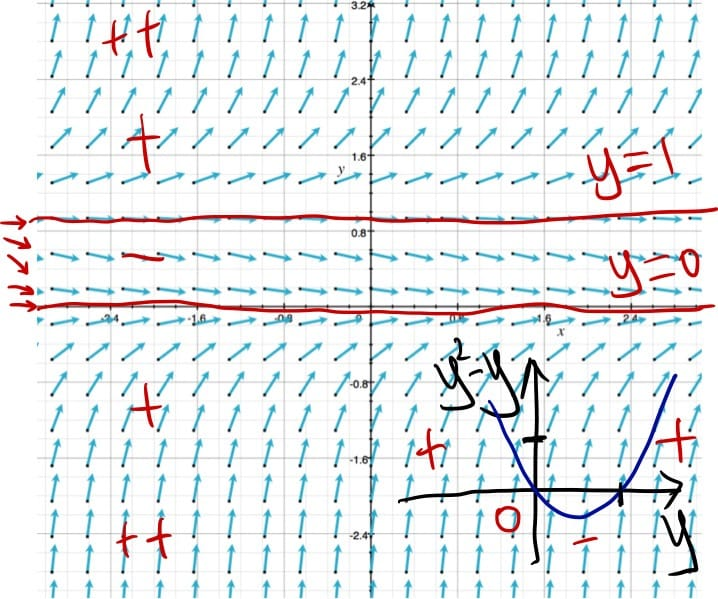5. 变换：可以用于任何函数，通过这种方法可以对函数有更直观的理解（非线性变换）。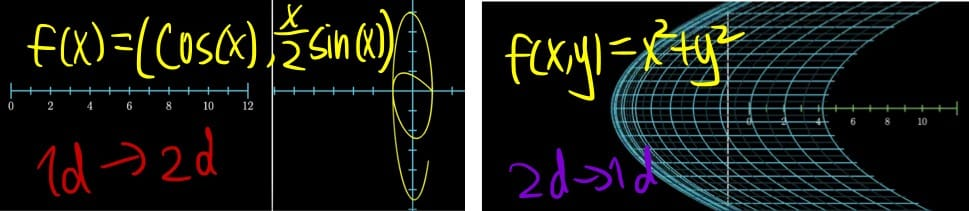多元函数的导数

偏导数（Partial Derivatives）

In fact, this intuitive feel for the symbol is one of the most useful takeaways from single-variable calculus, and when you really start feeling it in your bones, most of the concepts around derivatives start to click. 直观的理解很重要！

$\frac{\partial f}{\partial x}$（”$\partial$” 读作：/del/ or partial）：tiny change of $f$ with respect to tiny change of $x$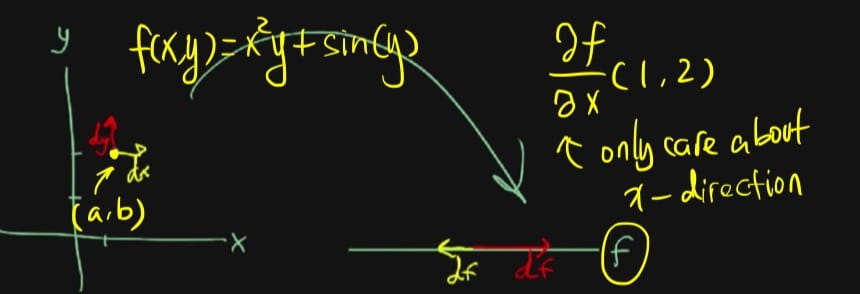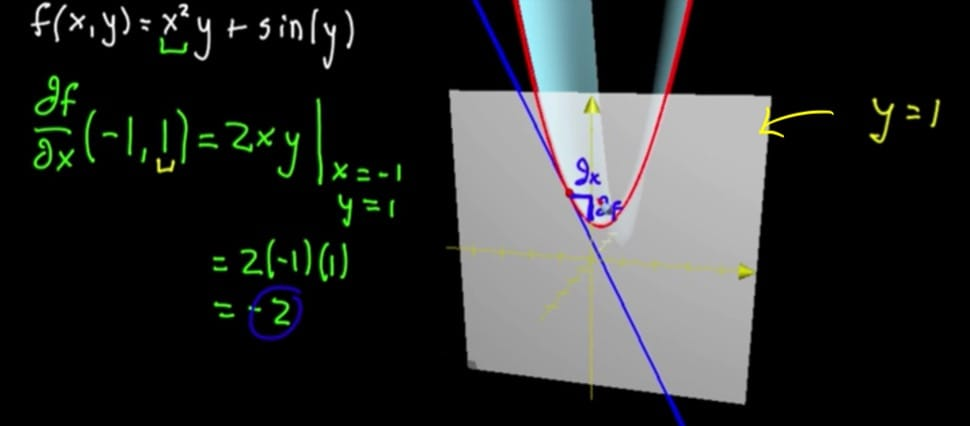梯度和方向导数（Gradient & Directional Derivatives）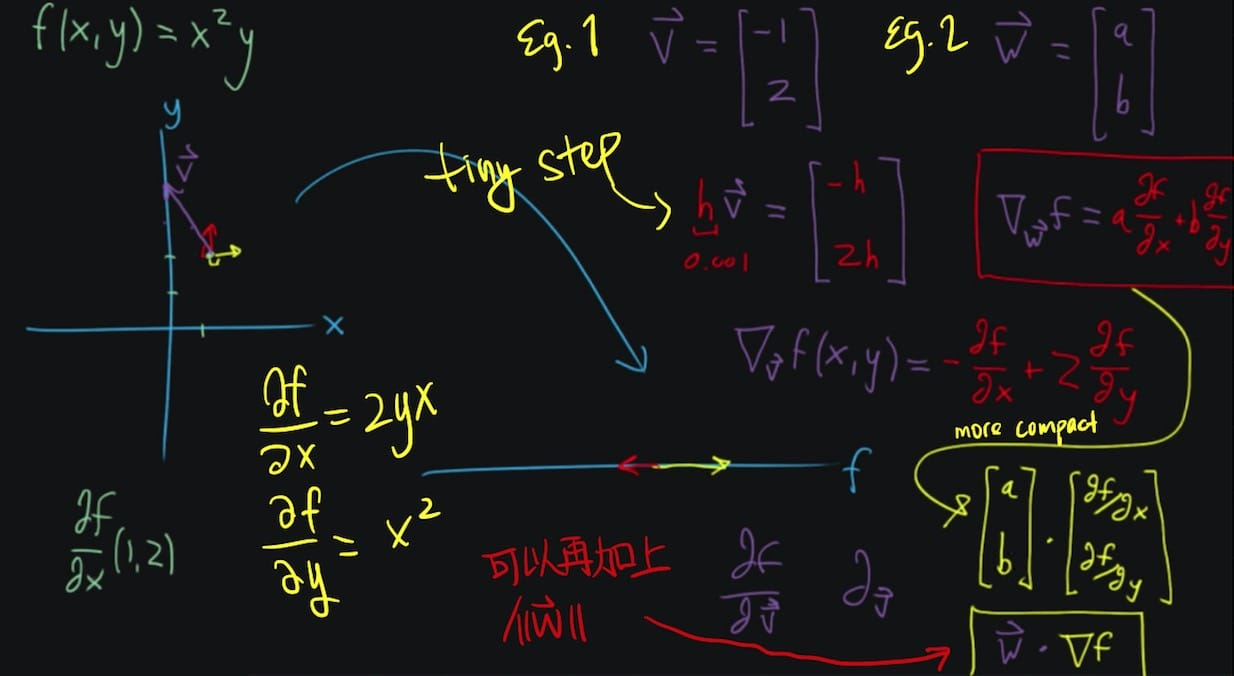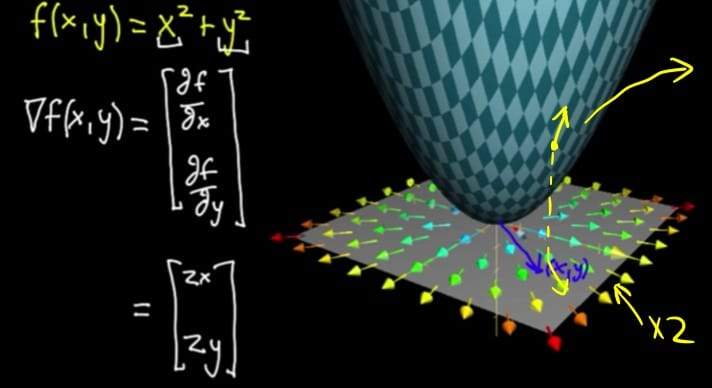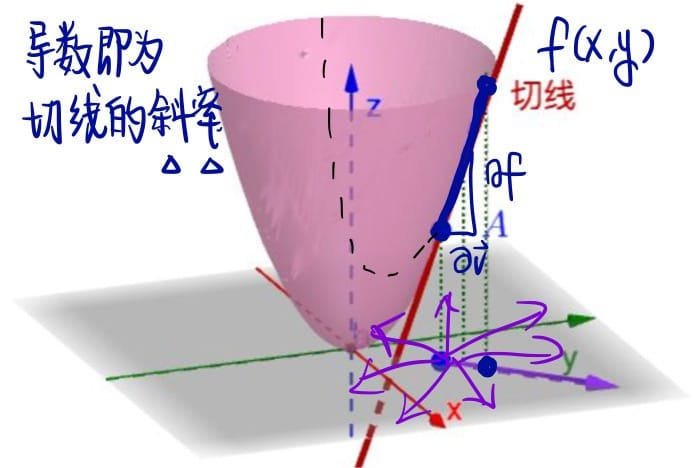$\nabla$ 可以看作是一个操作算子（Operator），其输入和输出均是函数 $f$，与 $\frac{d}{dx}$、$\frac{\partial}{\partial x}$ 类似。

• 计算方向导数时要不要将向量归一化？

If the directional derivative is used to compute slope, either $\vec{v}$ must be a unit vector or you must remember to divide by $||\vec{v}||$ at the end. Then you will get $\nabla_{\vec{v}\ }f(\vec{x})=\lim\limits_{h\rightarrow0}\frac{f(\vec{x}+h\vec{v})-f(\vec{x})}{h||\vec{v}||}$.
However, this definition puts too much emphasis on the particular use case of finding slope, so I (not me…) prefer to use the original definition and normalize $\vec{v}$ when necessary.

• 为什么只有一个最大值（方向导数）？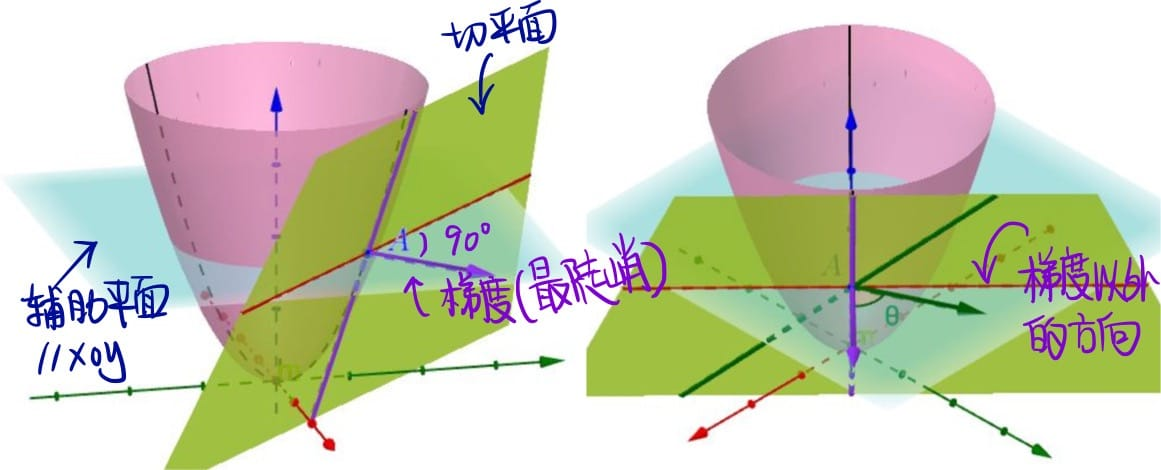对参数曲线微分❓（Differentiating Parametric Curves）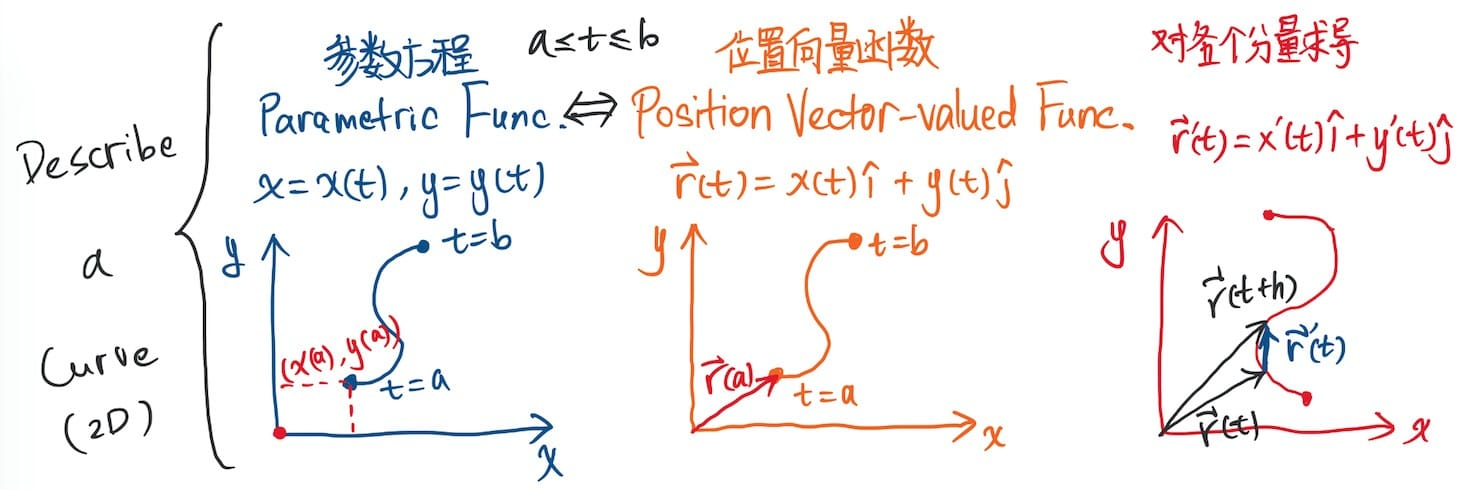多元链式法则（Multivariable Chain Rule）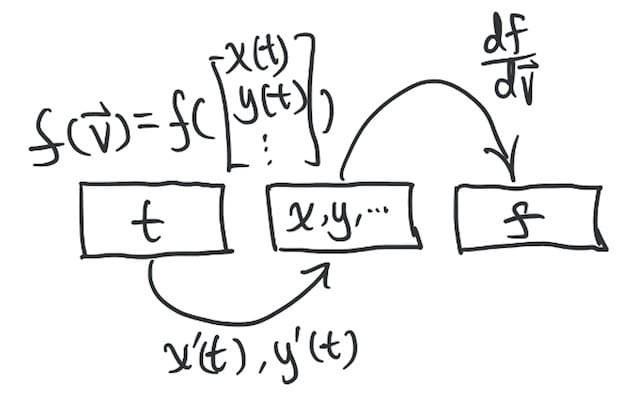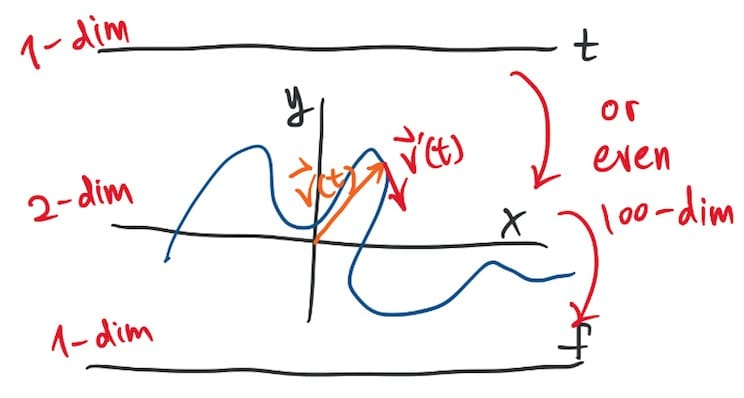其它概念

曲率（Curvature）

how to describe how much you turn?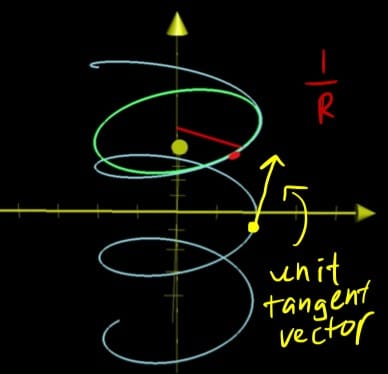散度（Divergence）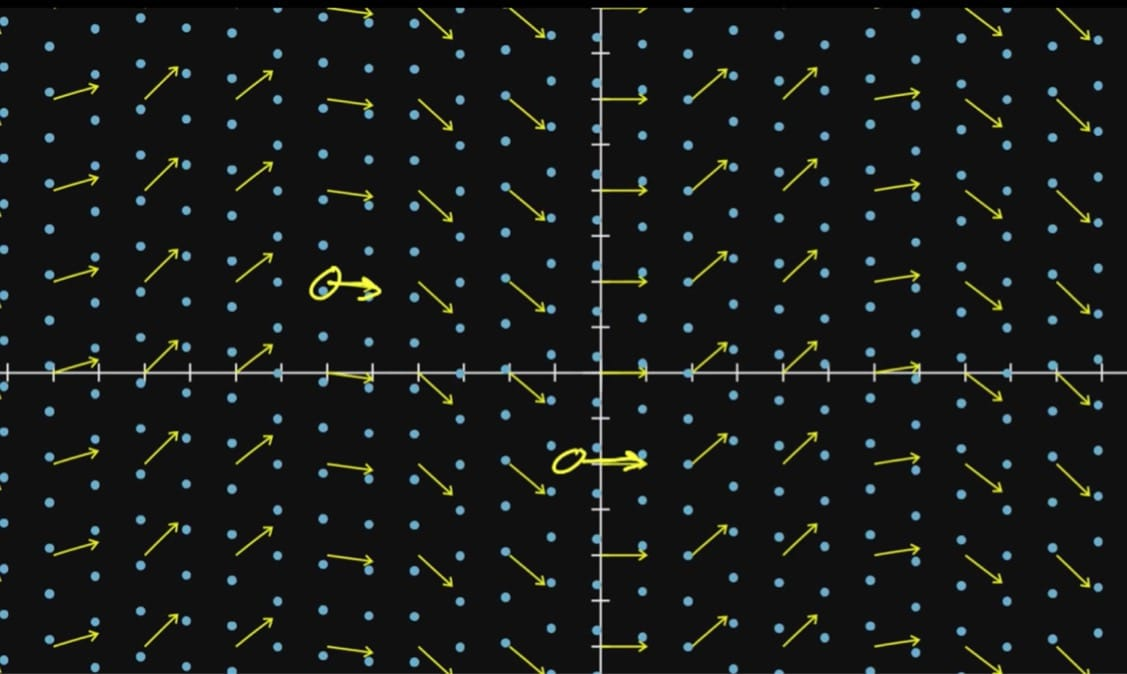旋度（Curl）

Curl measures the rotation in a fluid flowing along a vector field. Formally, curl only applies to 3D, but here we cover the concept in 2D to warmup.

2D 下不正式的旋度计算：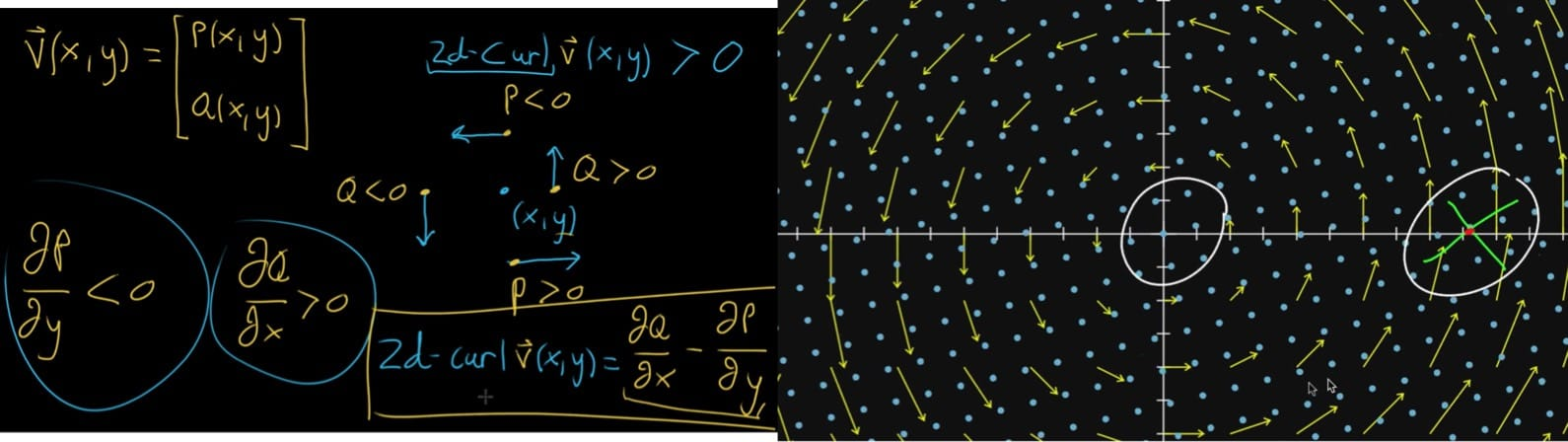3D 下的定义：可以表示三维向量场对某一点附近的微元造成的旋转程度。向量场每一点的旋度是一个向量，称为旋度向量，可表示为（运用了Notational Trick）：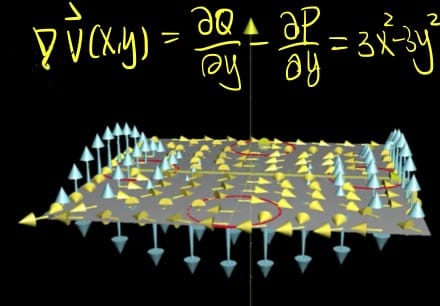Interesting：旋度向量的大小等于改点上角速度的两倍。

拉普拉斯算子（Laplacian）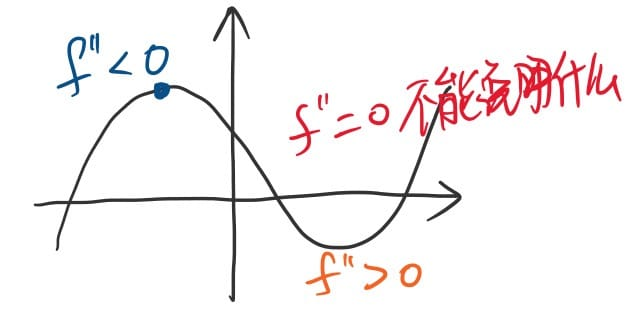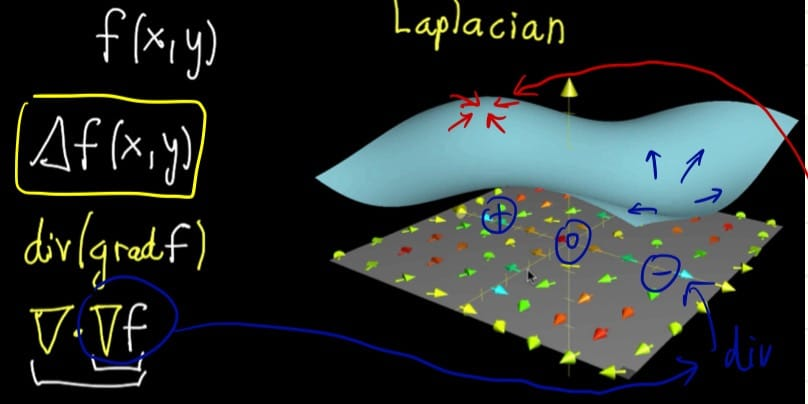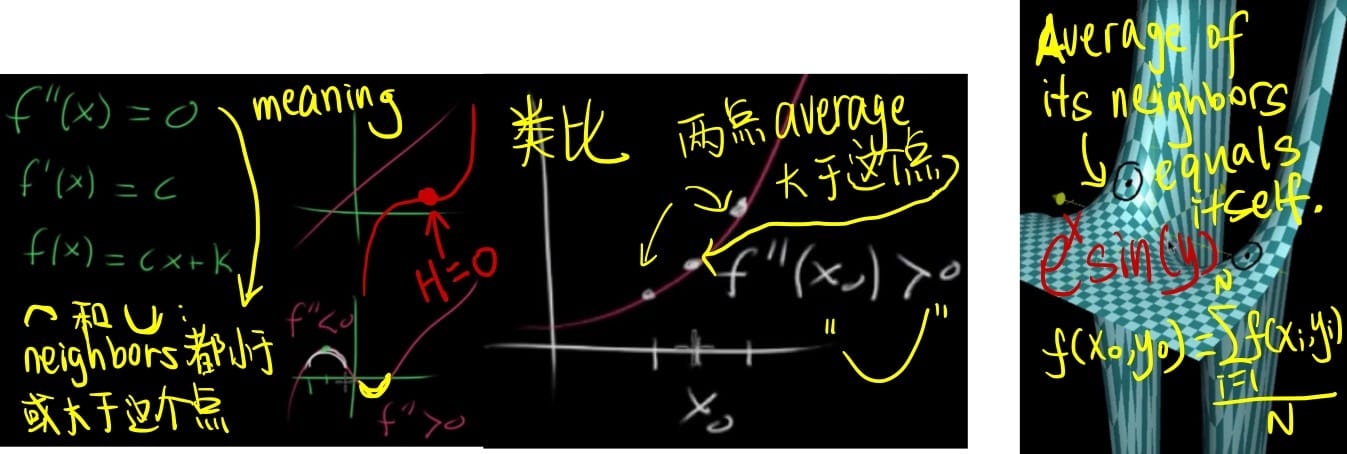雅克比矩阵（Jacobian Matrix）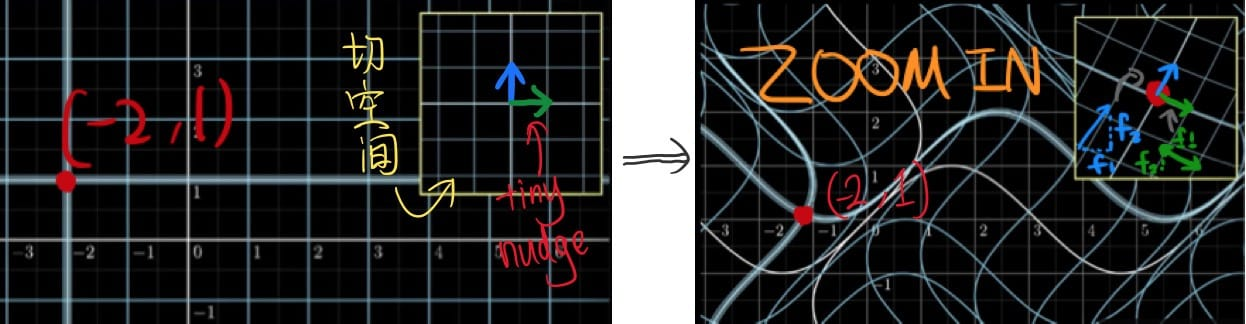多元导数的应用

局部线性化逼近（Local Linearization）

The idea is to approximate a function near one of its inputs with a simpler function that has the same value at that input.

2D 下的几何意义：如何控制这个切平面，如图所示。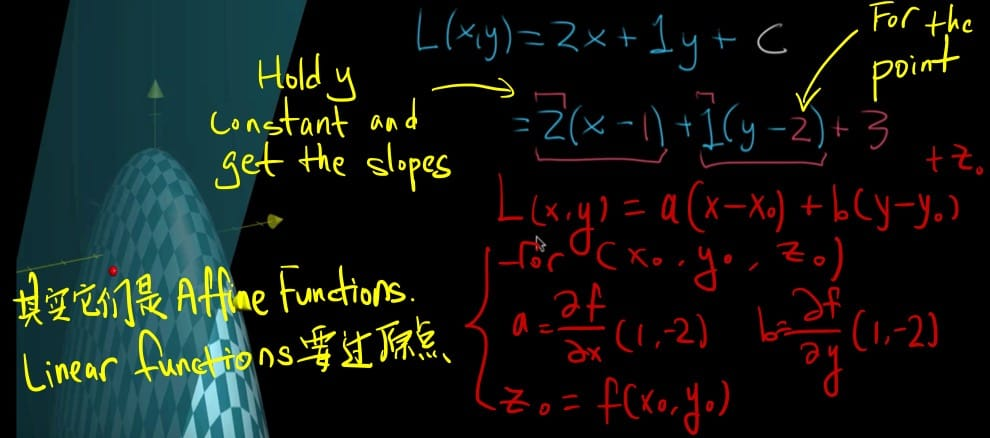“Quadratic” means product of two variables. $x_0$ is read “x naught”.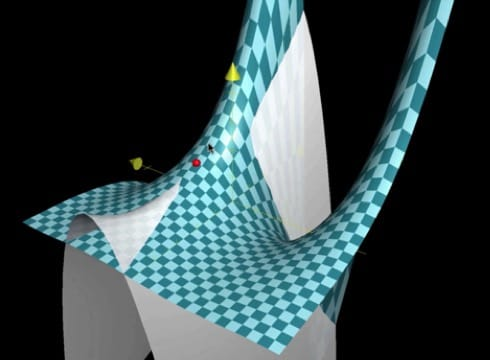• 函数值相等，$f(x,y)=Q(x,y)$

• 一阶偏导都相等，$f_x=Q_x$、$f_y=Q_y$

• 二阶偏导都相等，$f_{xx}=Q_{xx}$、$f_{yy}=Q_{yy}$、$f_{xy}=Q_{yx}$

• 一元：$\frac{df}{dx}$、$\frac{d^2f}{dx^2}$、$\frac{d^3f}{dx^3}$、…

• 多元：$\nabla{f}$、$H_f$、$\cal{Multilinear\ Algebra}$❓

多元函数的最优化（Optimizing Multivariable Functions）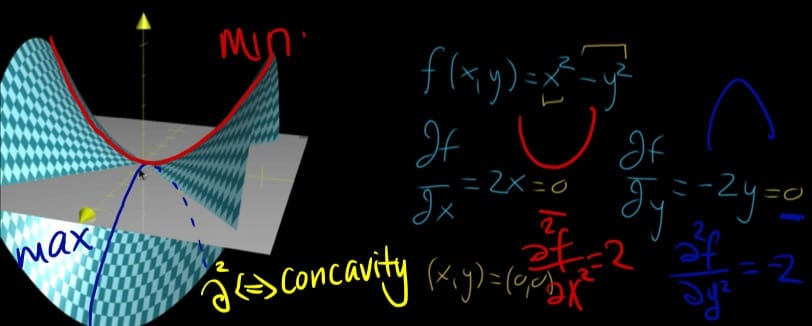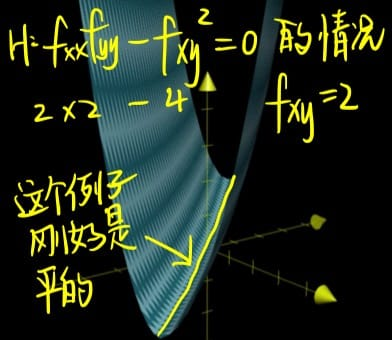• 当 $f’(a)=0$ 时，$f(x)\approx f(a)+\frac{1}{2}f’’(a)(x-a)^2$，因为 $(x-a)^2\geq 0$，所以 $f’’(a)$ 可以决定局部极小值和局部极大值。

• 当 $f’(a)=0$、$f’’(a)=0$ 时，$f(x)\approx f(a)$ 得到的是一个常量，无法决定是局部极大值还是局部极小值。

拉格朗日乘子法和约束优化（Lagrange Multipliers $\lambda$ and Constrained Optimization）

• 收入（Revenue）：$R(x,y)=x^2e^yy$

• 预算/成本（Budget）：$B(x,y)=x^2+y^2$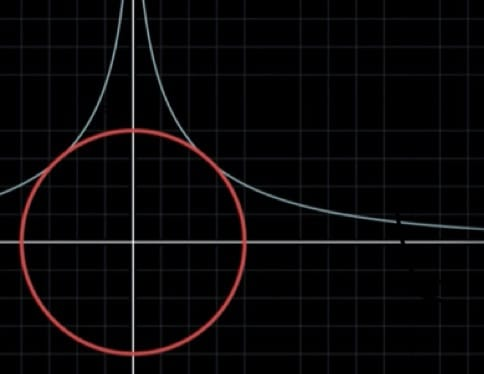$\lambda$ 有什么含义：

$\cal{L}^*$ 和 $\cal{L}$ 不同，前者是四元函数（包含 $b$），后者是三元函数。

KKT 条件：马住❗️

0%